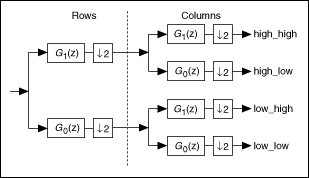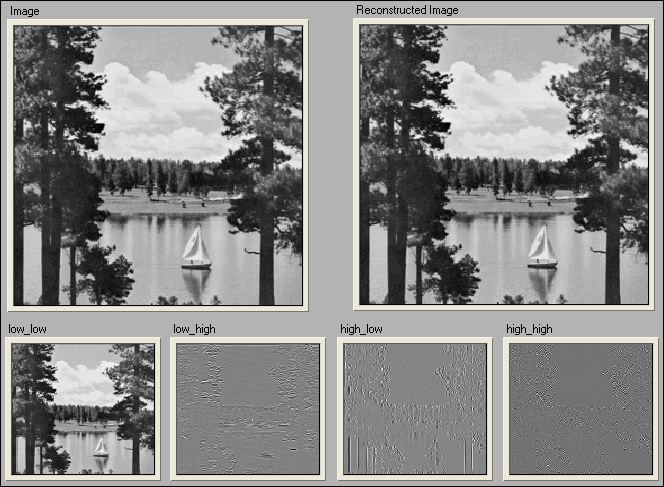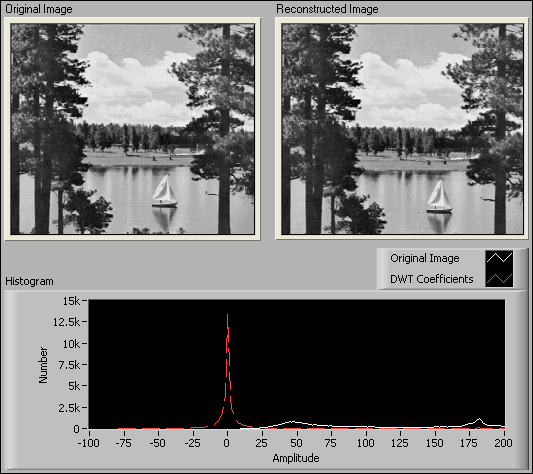# 2D Signal Processing (Advanced Signal Processing Toolkit)

LabVIEW 2014 Advanced Signal Processing Toolkit Help

Edition Date: June 2014

Part Number: 372656C-01

»View Product InfoDownload Help (Windows Only)

Using the LabVIEW Wavelet Analysis Tools, you can extend the discrete wavelet transform (DWT) to 2D signal processing.

The following figure shows the PR filter bank implementation of the 2D DWT, which applies the filter banks to both rows and columns of an image.As the previous figure shows, when decomposing 2D signals with two-channel PR filter banks, you process rows first and then columns. Consequently, one 2D array splits into the following four 2D arrays:

• low-low
• low-high
• high-low
• high-high

Each array is one-fourth of the size of the original 2D array.

The following figure shows an example of decomposing and reconstructing an image file with the 2D DWT and the inverse 2D DWT.The source image decomposes into the following four sub-images:

• low_low—Shows an approximation of the source signal with coarse resolution.
• low_high—Shows the details at the discontinuities along the column direction.
• high_low—Shows the details at the discontinuities along the row direction.
• high_high—Shows the details at the discontinuities along the diagonal direction.

You can apply the decomposition iteratively to the low_low image to create a multi-level 2D DWT, which produces an approximation of the source signal with coarse resolution. You can determine the appropriate number of decomposition levels for a signal-processing application by evaluating the quality of the decomposition at different levels.

Use the Multiresolution Analysis 2D Express VI to decompose and reconstruct a 2D signal.

The following figure shows an example of image compression using the 2D DWT with the FBI wavelet.The histogram of the DWT Coefficients plot shows that the majority of the DWT coefficients are small, meaning that you can use a small number of large DWT coefficients to approximate the image and achieve data compression.

Not Helpful